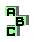ANTIPEDAL TRIANGLE

 r25 Fermat points, equilateral triangle r338 circumcenter, perspective r381 pedal triangle, isogonal points, homothetic triangles r441 centroid, Steiner inellipse r494 Vecten squares, centroid, point X(376), homothetic triangles r729 point X(376), centroid r1107 cevian triangle, anticevian triangle, orthologic triangles r1221 circumcenter, circumcevian triangle, perspective r1460 isogonal points r1467 isogonal points, orthologic triangles r1667 centroid, Fermat points r1764 antigonal points, medial triangle, circumcenter, Parry reflection point, perspective, homothetic triangles r1876 ninecenter, circumcenter, complement r1964 isogonal points r2040 incenter, circumconic, incentric triangle, Spieker point, orthologic triangles r2050 orthologic triangles